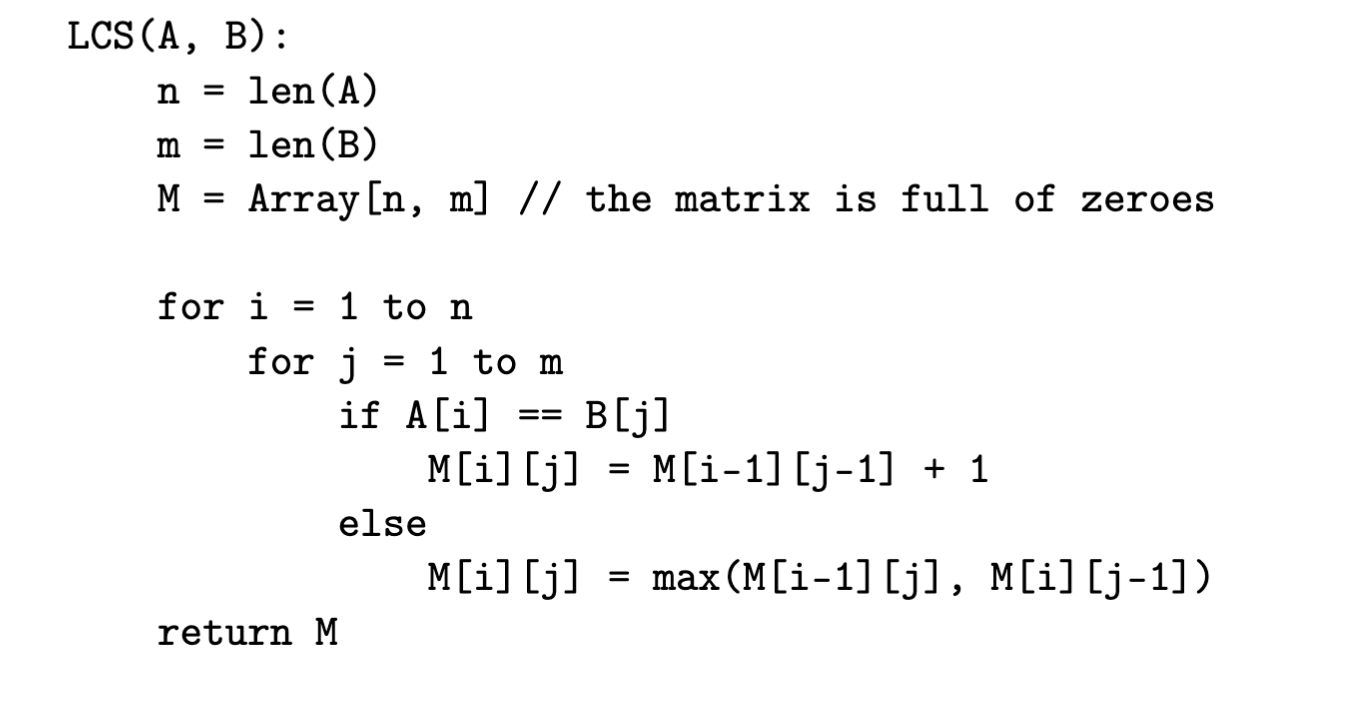### L - LCS Recovery

##### Time & Memory limits:(details)

The LCS (Longest Common Subsequence) algorithm of two binary strings $A$ and $B$ returns a matrix as follows:Given a matrix $M$, find the smallest among all possible pairs of binary strings $A$ and $B$ such that LCS(A, B) returns $M$. A pair $(A, B)$ is smaller than $(C, D)$ if $(A+B)$ is lexicographically smaller than $(C+D)$ where the operator $+$ denotes standard string concatenation.

#### Input

First line of the input contains two integers $n$ and $m$ $(1 \le n, m \le 2 \cdot 10^3)$, the number of rows and the number of columns in the matrix.

Next $n$ lines contain $m$ integers each. The $j$-th element in the $i$-th line is $M[i][j]$.

It is guaranteed that there exists at least two binary strings such that the LCS algorithm returns the given matrix.

Please note that the given matrix differs from the one at the pseudocode by not having the row number 0 and column number 0 for simplicity.

#### Output

Print one binary string $A$ of length $n$ on the first line and one binary string $B$ of length $m$ on the second line, such that the pair $(A, B)$ is the smallest lexicographically where the LCS algorithm returns the given matrix.

#### Sample test(s)

Input
2 3 0 1 1 0 1 2
Output
00 100
Input
3 4 0 1 1 1 0 1 2 2 0 1 2 3
Output
000 1000
Input
5 5 0 0 1 1 1 1 1 1 1 2 1 2 2 2 2 1 2 2 2 3 1 2 2 2 3
Output
01111 11001